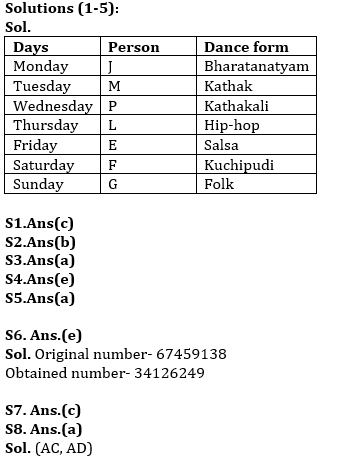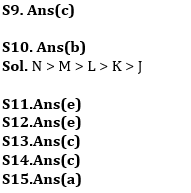Latest Banking jobs   »   Reasoning Ability Quiz For ESIC- UDC,...

# Reasoning Ability Quiz For ESIC- UDC, Steno, MTS Prelims 2022- 28th January

Directions (1-5): Study the information carefully and answer the questions given below.
Seven persons are going on attend dance class on seven different days of the week in such a way week starts from Monday. Each of them likes different dance forms i.e. Kathak, Salsa, Folk, Bharatanatyam, Kathakali, Kuchipudi and Hip-hop but not necessary in the same order.
P likes kathakali and attends class on Wednesday. One person attends class between P and J. The one who likes salsa dance attend class on Friday. Two persons attend class between the one who likes salsa and the one who likes Kathak. More than three persons attend class between J and F. G attends class on Sunday. The one who likes Kuchipudi dance attends class before the one who likes Folk dance and after the one who likes hip-hop dance. J does not like hip-hop dance. M attend the class before E. L likes Hip-hop dance.

Q1. Who among the following likes salsa dance?
(a) P
(b) M
(c) E
(d) L
(e) None of these

Q2. Who among the following person like Kuchipudi dance?
(a) E
(b) F
(c) L
(d) G
(e) None of these

Q3. How many persons attend class between the one who likes folk dance and P?
(a) Three
(b) Two
(c) Four
(d) One
(e) None

Q4. Which of the following combination is true?
(a) M- Hip-hop
(b) L-Salsa
(c) E-Kuchipudi
(d) G-Bharatanatyam
(e) None is true

Q5. How many persons attend the class before the one who likes Bharatanatyam?
(a) No One
(b) Two
(c) Three
(d) One
(e) Four

Q6. If in the number 67459138, 3 is subtracted to first five digit of the number and 1 is added to rest of the digits then how many digits are repeating in the number thus formed?
(a) Four
(b) One
(c) None
(d) three
(e) Two

Q7. Which of the following elements should come in a place of ‘?’
GJ11   MO16   RT21   ?
(a) WX26
(b) VX25
(c) WY26
(d) VY26
(e) None of these

Q8. How many pairs of letters are there in the word “ENDURANCE” each of which have as many letters between them in the word as they have between them in the English alphabetical series?
(a) Two
(b) One
(c) Three
(d) More than three
(e) None

Q9. Ravi is 22nd from the left end of a row and Hunny is 32th from the right end of row. If they interchanged their positions then ravi ranks become 21 from left end. How many persons sit between them?
(a) One
(b) Two
(c) None
(d) Three
(e) None of these

Q10. Among J, K, L, M and N, each of them is different weight. L’s weight is more than K. M’s weight is more than J and less than N. K is not the lightest person. M is not lighter than L. Who among them is the third heaviest?
(a) J
(b) L
(c) K
(d) M
(e) N

Directions (11-15): Following questions are based on the five three-digit numbers given below.

947   376   694   739   863

Q11. If all the digits in each of the numbers are arranged in increasing order within the number then, which of the following number will become the lowest in the new arrangement of numbers?
(a) 947
(b) 863
(c) 739
(d) 694
(e) 376

Q12. If all the numbers are arranged in ascending order from left to right then, which of the following will be the sum of all the three digits of the number which is 2nd from the right in the new arrangement?
(a) 18
(b) 19
(c) 15
(d) 16
(e) None of these

Q13. What will be the difference, when third digit of the 3rd lowest number is multiplied with the second digit of the highest number and third digit of the 2nd highest number is multiplied with the second digit of the lowest number?
(a) 21
(b) 20
(c) 15
(d)16
(e) None of these

Q14. If the positions of the second and the third digits of each of the numbers are interchanged then, how many even numbers will be formed?
(a) None
(b) One
(c) Two
(d) Three
(e) Four

Q15. If one is added to the second digit of each of the numbers and one is subtracted to the third digit of each number then, how many numbers thus formed will be divisible by three in new arrangement?
(a) None
(b) One
(c) Two
(d) Three
(e) Four

Solutions#### Congratulations!